## Crack propagation

A cyclic crack propagation calculation consists of a series of increments. Each increment is considered to be independent of the others. The input for an increment is characterized by stresses and maybe temperatures for the uncracked structure, a triangulation of the current cracks using S3 shell elements and crack propagation material data. The following operations are performed before the first increment:

1. read the crack propagation material data
2. read and store the uncracked stress and possibly temperature results in fields integerglob and doubleglob

Subsequently, in each iteration the following actions are taken:

1. catalogue the nodes belonging to the field kontri(3,1..ntri) where ntri is the total number of triangles in all cracks (cattri.f).
2. catalogue the edges in fields ipoed(1..nk), iedg(3,1..nedg) and ieled(2,1..nedg), where nk is the total number of nodes in the model and nedg the total number of edges belonging to S3-elements. An edge is characterized by two nodes and is catalogue according to the lowest node number of both. Entry ipoed(n1) points to a row iedg(1..3,ipoed(n1)), in which n1=iedg(1,ipoed(n1)) is one of the nodes and n2 = iedg(2,ipoed(n1))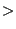n1 is the other node. If more edges are present in the mesh for which node n1 is the lowest node number, iedg(3,ipoed(n1)) points to the next such entry in iedg, else iedg(3,ipoed(n1)) is zero. This is similar to the structure on the left of Figure 182, except that in the present context the number of edges is not changed within an increment and the flag ifreeed is not needed. For each edge i the entries ieled(1,i) and ieled(2,i) point to the triangle numbers in kontri to which the edge belongs. For an edge belonging to only 1 triangle entry ieled(2,i) is zero.
3. determine the boundary edges and the boundary nodes. An edge is a boundary edge if it belongs to only one triangle. A node is a boundary node if it belongs to a boundary edge. The boundary nodes are stored in field ibounnod(1..nbounnod), the boundary edges in ibounedg(1..nbounedg). The field iedno(2,1..nbounnod) contains the boundary edge numbers to which a boundary node belongs. It is important to distinguish between the edge numbers (1...nedg), correspondng to the rows in field iedg, and the boundary edge numbers (1....nbounedg), corresponding to the rows in field ibounedg. The fields ibounnod and ibounedg together with some other fields, which will be discussed soon, are shown in Figure 190. The nodes in field ibounnod are sorted in ascending order.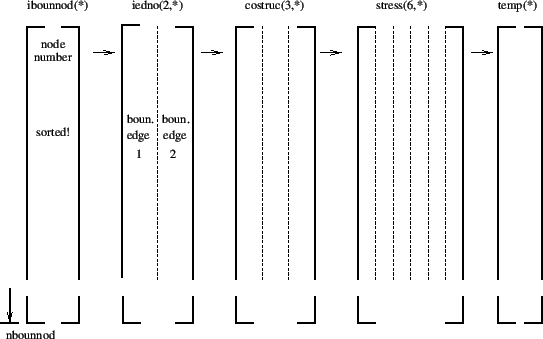4. determine the front nodes: these are boundary nodes (i.e. on the boundary of the crack triangulations) lying inside the structure. The way this is done is by taken recourse to routines interpolextnodes.f and basis.f. The latter routine interpolates the stress and temperature from the uncracked structure onto each boundary node. In fact, basis.f is a very general routine doing the interpolation to whatever point characterized by its global carthesian coordinates. It looks for a location inside the master mesh which is as close as possible to the given point and assigns the fields interpolated in this location. Furthermore, it returns the interpolated values, the interpolation coefficients, the nodes of the master mesh used for the interpolation, the coordinates of the interpolation location and the distance from the given point to the interpolation location. If this distance is really small (a cut-off of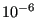is used), the boundary node is a front node, else it is not. The front nodes are stored in ascending order in field ifront(1..nfront), the corrosponding boundary node location (i.e. the row in field ibounnod) is stored in ifrontrel(1..nfront), cf. Figure 191. The coordinates of the interpolation locations are stored in costruc(3,1..nbounnod) and the interpolated stresses and temperatures in stress(6,1..nbounnod) and temp(1..nbounnod), cf. Figure 190.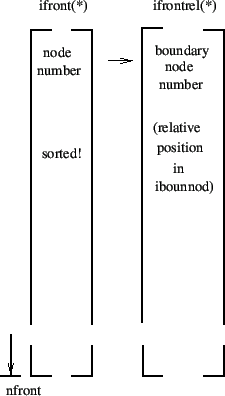Notice that when using the coordinates in field costruc one obtains a contour of the crack contracted on the structure, i.e. the boundary nodes outside the structure are projected onto the structure.

5. determine the due order of the nodes in field ifront by taking the adjacency relations into account (done in routine adjacentbounodes.f) and adding to each non-closed front a node on either side (start and end of the front) just outside the structure, Figure 192. Due to this, the value of nfront will have changed if not all cracks are subsurface cracks. The nodes are stored in clockwise direction when looking in the positive shell normal direction. The start and end location of each front is stored in fields istartfront(1..nnfront) and iendfront(1..nnfront), where nnfront is the number of fronts. A zero in the corresponding field isubsurffront(1..nnfront) indicates that the front belongs to a surface crack, a one that it belongs to a subsurface crack, Figure 193. The field “field” in Figure 192 is representative for a large number of fields: xt(3,*), xn(3,nstep,*), xa(3,nstep,*), xnplane(3,nstep,*), xaplane(3,nstep,*), posfront(*), acrack(nstep,*), xk1(nstep,*), xk2(nstep,*), xk3(nstep,*), xkeq(nstep,*), phi(nstep,*), psi(nstep,*), xkeqmin(*), xkeqmax(*), dadn(*), wk1(*), wk2(*), wk3(*), dkeq(*), domstep(*), domphi(*), ifrontprop(*), which will be discussed further in this section.

The fronts are stored crack by crack. The start and end of each crack in field ifront is stored in fields istartcrackfro(1..ncrack) and iendcrackfro(1..ncrack) (Figure 194), where ncrack is the number of cracks. Figure 192 applies to a case in which the first crack consists of two fronts.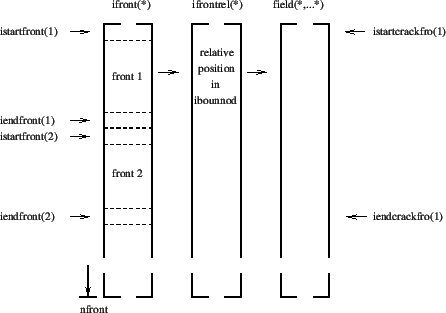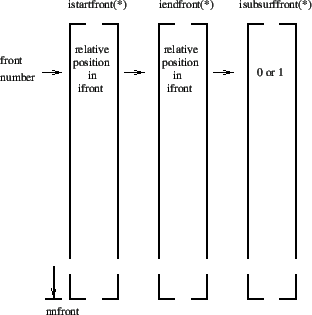At the same time, also the node order in field ibounnod is changed according to adjacency, crack by crack. Remember that this field contains all boundary nodes, no matter whether they belong to a front or not. Therefore, each crack in field ibounnod is a closed contour. After changing the node order in ibounnod all related fields in Figure 190 are also modified appropriately as well as the entries in field ifrontrel (Figure 192), so that full consistency is guaranteed. The starting and ending position of each crack in field ibounnod is stored in istartcrackbou(1..ncrack) and iendcrackbou(1..ncrack).

6. A local coordinate system in each node of the crack front is created and stored in fields xt(3,nfront), xn(3,nfront) and xa(3,nfront). This is done in routine createlocalsys.f. It consists of:
• A local normalized tangent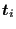(field xt) to the crack front (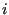is the nodal position).
• a normal vector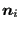obtained by taking the mean of the normal vectors on the shell elements to which the front node belongs, projecting this mean vector onto a plane orthogonal to the local tangent and normalizing. Notice that this normal vector is pointing in the positive direction of the shell elements (field xn).
• a normalized vector in propagation direction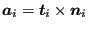(field xa).

7. Subsequently, the crack length is calculated in subroutine cracklength.f. In Section 6.9.25 the two different ways of calculating the crack length are explained: CUMULATIVE and INTERSECTION.
• The method CUMULATIVE is trivial to code
• The method INTERSECTION creates in each front node by means of the tangential vector a plane locally orthogonal to this vector and checks what other edge (but not immediately adjacent) along the closed crack front is cut by this plane (using fields such as ibounedg). An edge is cut by a plane if the substitution of the coordinates of its nodes into the plane equation yields results with a different sign.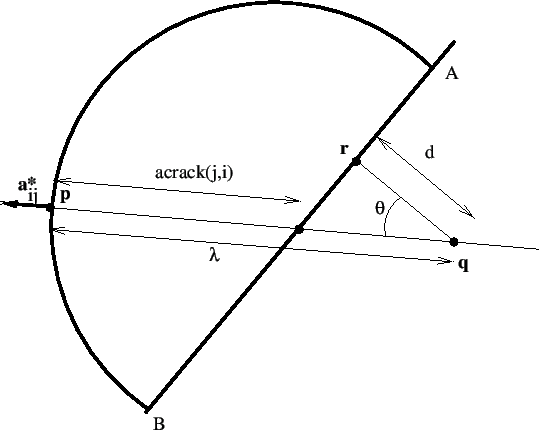The crack length is stored in field acrack(nfront). In addition, for each front node the relative position along the front, taking a value between 0 and 1, is also calculated in cracklength.f and stored in field posfront(nfront).

8. The crack length is smoothed and shape factors are determined in routines cracklength_smoothing.f and crack shape.f, respectively. The procedures are described in Section 6.9.25. For the shape factors a field shape(3,nfront) is created, allowing for different shape factors according to the mode. Routine shape.f is conceived as a user subroutine, so the user can easily code his/her own shape factors.

9. The stress intensity factors for mode I, mode II and mode III are calculated in stressintensity.f and stored in fields xk1(nstep,nfront), xk2(nstep,nfront) and xk3(nstep,nfront). The equivalent stress intensity factor, the deflection angle and twist angle are stored in xkeq(nstep,nfront), phi(nstep,nfront) and psi(nstep,nfront). Subsequently, the equivalent K-factor and the deflection angle is smoothed in stressintensity_smoothing.f. The target crack increment length is determined in calcdatarget.f. For details the reader is again referred to Section 6.9.25.

10. The crack propagation rate is calculated in routine crackrate.f. Again, this routine is conceived as a user subroutine. Right now, a rather simple algorithm is implemented using the maximum equivalent K-factor for the complete mission at a given location along the crack front. More sophisticated procedures are conceivable using cycle extraction such as in . Output of this routine includes the crack propagation increment da(nfront), the crack propagation rate dadn(nfront), the worst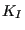,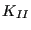and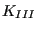factors wk1(nfront), wk2(nfront) and wk3(nfront), the largest and smallest equivalent K-factor in the mission xkeqmin(nfront) and xkeqmax(nfront), the equivalent stress intensity range of the main cycle dkeq(nfront), the dominant step dompstep(nfront) and the dominant deflection angle domphi(nfront). The number of cycles in this increment is calculated based on the target crack increment and the maximum crack propagation rate anywhere along a crack front.

11. In crackprop.f the position of the propagated node is calculated for each front node. The propagation is in the direction of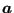. At a free boundary the new crack front has to cut the free surface, therefore at least the first and last node on a front have to lie outside the structure. This is checked by the interpolation routine basis.f. If e.g. the first node is inside the structure, the line segment connecting the node with its propagated position is rotated in steps of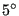until the propagated node lies outside the structure. In order for this procedure to work under all circumstances a minimum crack propagation increment of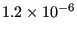[L] is defined.

Notice that for this procedure to work properly, the first and last node of the actual front have to lie on the intersection of the front with the free surface. This is not necessarily guaranteed by using the field costruc. Indeed, the values in costruc are determined in routine basis.f. If a node is inside the structure, costruc contains its actual coordinates, if it is outside the structure, it contains the coordinates of its projection onto the free surface. If the front makes an angle with the free surface which is significantly different from 90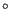, the projection will not lie on the front. Therefore, for the end nodes a correction is made similar to Figure 195: assuming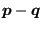is the crack front, the projected position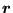is replaced by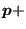acrack(i)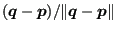.

The propagated node numbers are stored in ifrontprop(nfront).

12. Subsequently, on the new crack front consisting of the propagated nodes new equidistant nodes are created. The distance between these nodes is the mean distance between any two nodes on the initial front and is stored in charlen(1..nnfront). The equidistant nodes are stored in fields ifronteq(1..nfronteq), istartfronteq(1..nnfront) and iendfronteq(1..nnfront), which are completely analogous to ifront, istartfront and iendfront.

13. Finally, the mesh describing the crack is extended by the new increment. To this end new elements are generated connecting the nodes on on the actual front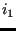,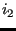, ...,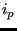with the propagated equidistance nodes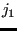,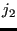, ...,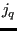. First, for each node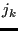the node among the actual nodes,.... is looked for which is the closest neighbor (using routine near3d.f). Let us call this node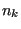. Then, the algorithm for the triangulation is as follows:

• set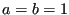• start loop
• create a triangle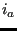,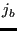and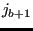.
• If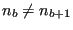, then let's say that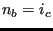(c naturally exists, since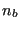belongs to the actual front). Then, create triangle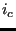,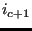,, triangle,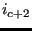,.. until triangle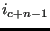,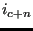,where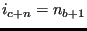. Set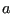to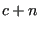.
• ifandboth belong to the last created triangle, exit.
• set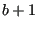to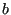.
• cycle loop

Notice that shell elements are expanded in CalculiX. Therefore, at the start of routine crackpropagation.c the crack surfaces are really modeled by 6-node wedge elements. Therefore, the extension of the crack is also modeled by wedges with a very small thickness.

14. store the incremental results into global fields (wk1glob,....)

15. start a new increment

After all increments have been calculated (or if nowhere along the crack front propagation occurred or if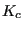was exceeded anywhere along the crack fronts) the results are stored in frd-format.